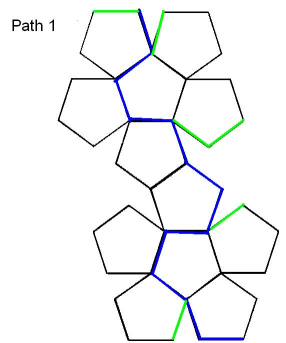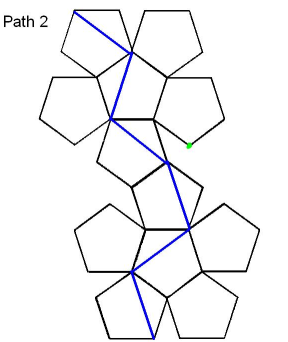#### You may also like### Just Rolling Round

P is a point on the circumference of a circle radius r which rolls, without slipping, inside a circle of radius 2r. What is the locus of P?### Coke Machine

The coke machine in college takes 50 pence pieces. It also takes a certain foreign coin of traditional design...### Just Opposite

A and C are the opposite vertices of a square ABCD, and have coordinates (a,b) and (c,d), respectively. What are the coordinates of the vertices B and D? What is the area of the square?

# Platonic Planet

##### Age 14 to 16Challenge Level

Elijah sent us a clear explanation of how he tackled this problem, along with some diagrams showing his solutions.

I made a dodecahedron and made my paths with string and Blu-Tac.
My first assumption was that Glarsynost was not extremely freakishly tall so she can't see over the horizon. Each edge is 1 flib long (a real alien word).

From the middle of a face she can see $\frac{1}{12}$ of the planet. From an edge she can see $\frac{1}{6}$ of the planet. From a vertex she can see $\frac{1}{4}$ of the planet.

FIRST PATH: Start at a vertex and keep going along a new edge so you can see a new face every time. The blue path can be seen in the picture - Path 1. Each face has at least one blue edge. The green lines show edges that are actually joined up to blue ones. The path is 12 flibs long.After that I wanted to cut across the faces so the path would be shorter and she could see new faces more quickly. So I wondered how long a diagonal of a pentagon is. My mum told me that it is $\frac{1 + \sqrt5}{2}$ flibs.

SECOND PATH: Start at a vertex and go across a diagonal so you can see two new faces. When you have seen them all, get back to the start. This is Path 2 in the picture. Each face has at least one blue vertex. The path is 6 diagonals long, $3(1 + \sqrt5)$ flibs which is about 9.7 flibs. This is the shortest path I could find, but there are other routes the same length.